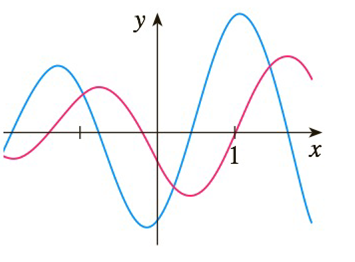Chapter 2.2, Problem 46E

Chapter
Section
Textbook Problem
5 views

# The graphs of a function f and its derivative f ' are shown. Which is bigger, f ' ( − 1 )  or  f ' ' ( 1 ) ?To determine

Which is bigger f'(-1)  or  f"(1)

Explanation

Given:

Wherever red graph has a horizontal tanget blue graph is crossing x-axis. Wherever red graph is decreasing blue graph is negative and also wherever red curve is increasing blue curve is positive. Thus we may conclud that blue curve is a graph of f' and red curve is a graph of f.

Now we see that the slope of tangent at x = -1 of the red curve is positive (because red curve is increasing at x = -1) that is  f'-1>0

### Still sussing out bartleby?

Check out a sample textbook solution.

See a sample solution

#### The Solution to Your Study Problems

Bartleby provides explanations to thousands of textbook problems written by our experts, many with advanced degrees!

Get Started

#### Find more solutions based on key concepts